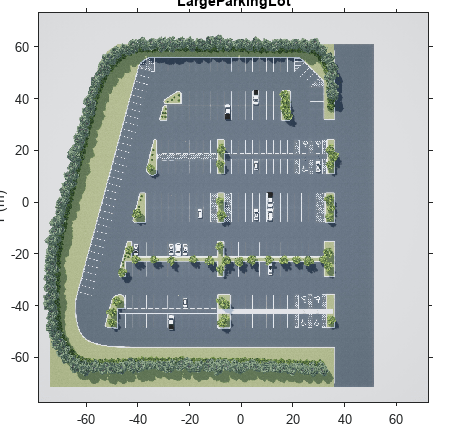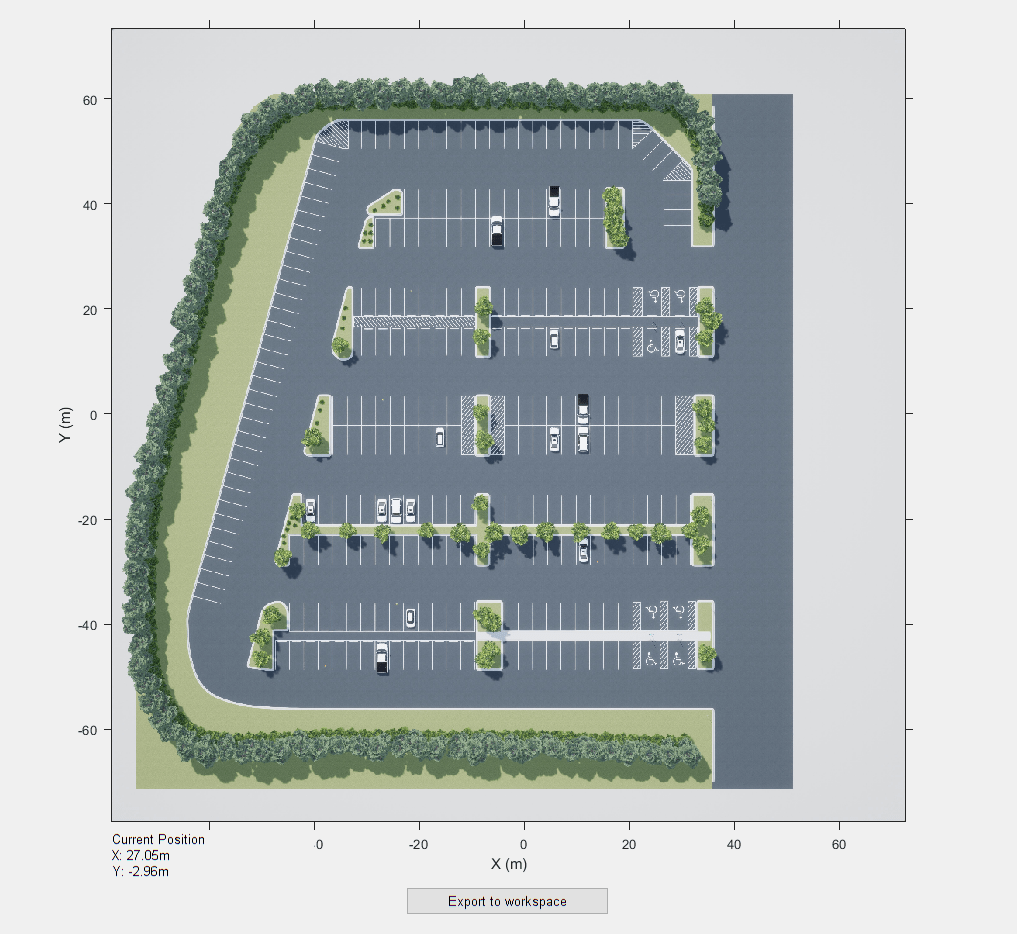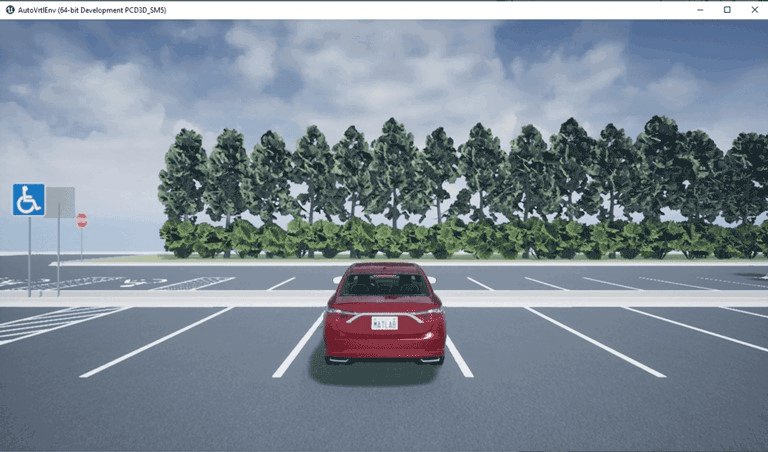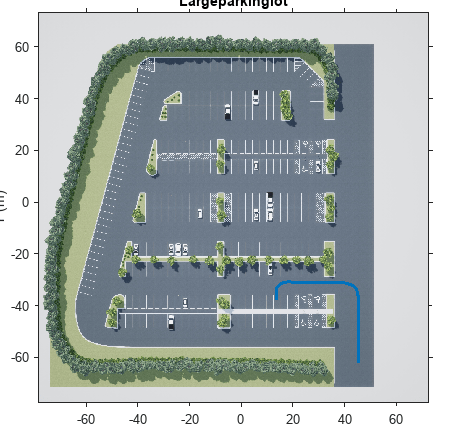# Select Waypoints for Unreal Engine Simulation

This example shows how to select a sequence of waypoints from a scene and visualize the path of a vehicle following these waypoints in a 3D simulation environment. This environment uses the Unreal Engine® by Epic Games®.

### Introduction

Automated Driving Toolbox™ integrates an Unreal Engine simulation environment in Simulink®. Simulink blocks related to the simulation environment can be found in the `drivingsim3d` library. These blocks provide the ability to:

• Select different scenes in the simulation environment.

• Place and move vehicles in the scene.

• Attach and configure sensors on the vehicles.

• Simulate sensor data based on the environment around the vehicle.

This powerful simulation tool can be used to supplement real data when developing, testing, and verifying the performance of automated driving algorithms. In conjunction with a vehicle model, you can use this environment to perform realistic closed-loop simulations that encompass the entire automated driving stack, from perception to control.

The first step in using this environment is understanding the scene and selecting waypoints along a desired vehicle path. This step is useful especially in scenarios where the localization algorithm is not under test. This example focuses on this first step.

In this example, you will:

• Visualize the scene in MATLAB®.

• Interactively select waypoints along a path in the scene.

• Set up the simulation environment.

• Move the vehicle along the path.

### Visualize Scene

First, visualize the scene in MATLAB. Each scene can be visualized using a 2D top-view projection of the scene onto an image. Each scene image has a corresponding 2D spatial referencing object of class `imref2d` describing the relationship between the pixels in the image and the world coordinates of the scene. Use the `helperGetSceneImage` function to retrieve the scene image and associated spatial reference. This example uses a prebuilt scene of a large parking lot. To generate a scene image and spatial reference for a custom scene, follow the process described in Create Top-Down Map of Unreal Engine Scene instead.

```sceneName = 'LargeParkingLot'; [sceneImage, sceneRef] = helperGetSceneImage(sceneName);```

To better understand the physical dimensions of the scene, inspect the `sceneRef` variable. The `XWorldLimits` and `YWorldLimits` properties specify the limits of the world in the X and Y directions.

`sceneRef.XWorldLimits % (in meters)`
```ans = 1×2 -78.6000 72.6000 ```
`sceneRef.YWorldLimits % (in meters)`
```ans = 1×2 -77.7000 73.5000 ```

Visualize the scene image by using the `helperShowSceneImage` function. This helper function displays the scene image in a figure window. Use the pan and zoom tools to explore the scene.

```hScene = figure; helperShowSceneImage(sceneImage, sceneRef) title(sceneName)```### Interactively Select Waypoints

After exploring the scene, select a set of waypoints to define a path for a vehicle to follow. This path can be used to move the vehicle in the scene. Use the helper function `helperSelectSceneWaypoints` to interactively select waypoints in the scene.

`hFig = helperSelectSceneWaypoints(sceneImage, sceneRef);`This helper function opens a figure window with the selected scene.

• Explore the scene by zooming and panning through the scene image. Use the mouse scrollwheel or the axes toolbar to zoom. Hover over the edge of the axes to pan in that direction.

• Begin drawing a path by clicking on the scene. A path is created as a polyline consisting of multiple points. Finish drawing the path by double-clicking or right-clicking.

• Once you are done drawing a path, click Export to Workspace to export the variables to the MATLAB workspace. In the dialog box that opens, click OK to export a set of variables to the workspace.

The following data is exported to the workspace as MATLAB variables:

• Waypoints: A cell array with each element containing an M-by-2 matrix of $\left(x,y\right)$ waypoints in world coordinates. Each element of the cell array corresponds to the waypoints from a different path.

• Path Poses: A cell array with each element containing M-by-3 matrices of $\left(x,y,\theta \right)$ poses containing the pose of each waypoint. $x$ and $y$ are specified in meters. $\theta$ is specified in degrees.

```% Load variables to workspace if they do not exist if exist('refPoses', 'var')==0 || exist('wayPoints', 'var')==0 % Load MAT-file containing preselected waypoints data = load('waypointsLargeParkingLot'); data = data.waypointsLargeParkingLot; % Assign to caller workspace assignin('caller', 'wayPoints', {data.waypoints}); assignin('caller', 'refPoses', {data.refPoses}); end```

The exported variables now contain a sequence of waypoints (`wayPoints`) and a sequence of poses (`refPoses`). Use the `smoothPathSpline` function to transform the sequence of poses to a ${C}^{2}$ continuous path.

```numPoses = size(refPoses{1}, 1); refDirections = ones(numPoses,1); % Forward-only motion numSmoothPoses = 20 * numPoses; % Increase this to increase the number of returned poses [smoothRefPoses,~,cumLengths] = smoothPathSpline(refPoses{1}, refDirections, numSmoothPoses);```

### Set Up Model and Simulation Environment

Open the `VisualizeVehiclePathIn3DSimulation` Simulink model. This model uses the Simulation 3D Scene Configuration block to select a desired scene. This example uses the Large Parking Lot scene. The Simulation 3D Scene Configuration block sets up the environment and establishes communication between Simulink and the simulation environment.

```if ~ispc error(['3D Simulation is only supported on Microsoft', char(174), ' Windows', char(174), '.']); end modelName = 'VisualizeVehiclePathIn3DSimulation'; open_system(modelName); snapnow;```

### Move Vehicle Along Path

Use the Simulation 3D Vehicle with Ground Following block to place and move vehicles in the scene. The model is set up to accept variables `refPosesX`, `refPosesY`, and `refPosesT` from the workspace using the From Workspace (Simulink) block. Separate $x$, $y$ and $\theta$ from `newRefPoses` into separate time series. The model reads these workspace variables to update the position of the vehicle.

```% Configure the model to stop simulation at 5 seconds. simStopTime = 5; set_param(gcs, 'StopTime', num2str(simStopTime)); % Create a constant velocity profile by generating a time vector % proportional to the cumulative path length. timeVector = normalize(cumLengths, 'range', [0, simStopTime]); % Create variables required by the Simulink model. refPosesX = [timeVector, smoothRefPoses(:,1)]; refPosesY = [timeVector, smoothRefPoses(:,2)]; refPosesT = [timeVector, smoothRefPoses(:,3)];```

When you simulate the model, a few seconds are needed to initialize the simulation environment. Once this initialization is complete, a separate window opens for the simulation environment visualization. The image below is a snapshot of the simulation environment window.Run the simulation. The figure window plot shows the path that the vehicle traverses through the simulation environment.

`sim(modelName);````% Close the model and figure windows. close(hFig) close_system(modelName) close(hScene)```